Get 1 free lesson per week // Add a new lessonNew account
Millions of accounts created on our sites.

• Home
• Print
• Guestbook
• Report a bug

•Get a free English lesson every week!

Partners:
- Our other sites

Learn English > English lessons and exercises > English test #3778: Fractions> Other English exercises on the same topic: Numbers [Change theme] > Similar tests: - Cardinal and ordinal numbers - Fractions and percentages - ESL-Numbers from 1 to 10 - Geometric shapes - Vocabulary: mathematics - Geometry-Vocabulary - Idioms: numbers - Numbers and dates... (Level 1) > Double-click on words you don't understand

# Fractions

A fraction is a part of a whole, like one half (1/2), one third (1/3), two thirds (2/3), one quarter (1/4), etc. For example, half of an apple is a fraction of an apple.

A fraction can be expressed in the form a/b, where the top number, a, is called the numerator; the bottom number, b, is called the denominator. The denominator of a fraction cannot be equal to zero (this is because you cannot divide a number by zero).

The value of a fraction is not changed if both the numerator and denominator are multiplied or divided by the same non-zero number.

Equivalent Fractions :Equivalent fractions are different fractions that are equal to the same number (like 2/4 and 3/6) and can be simplified and written as the same fraction (1/2 in this example).

Expressing Fractions in Simplest Form :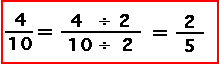A fraction is in its simplest form (this is also called being expressed in lowest terms) if the Greatest Common Factor (GCF), also called the Greatest Common Denominator (GCD), of the numerator and denominator is 1. To reduce a fraction to lowest terms (also called its simplest form), divide both the numerator and denominator by the GCD. For example, 2/3 is in lowest form, but 4/6 is not in lowest form (the GCD of 4 and 6 is 2) and 4/6 can be expressed as 2/3.

Improper Fractions :An improper fraction is one whose numerator is larger than the denominator (like 5/4). Improper fractions can be written as a mixed number, a number plus a fraction (for example, 5/4 = 1 1/4).

Fraction Written as Decimals :Every fraction can be expressed as decimal (a number with a decimal point). One way to convert a fraction to a decimal is to divide the numerator by the denominator. For example, 1/2 is equal to 1 divided by 2, which is equal to 0.5.

Fractions to Percents :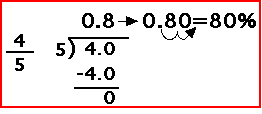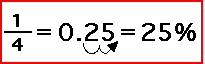A fraction can be expressed as a percentage by converting the fraction to a decimal number, then multiplying by 100 to obtain a percentage (since percent means per 100). For example, 1/2 = 0.5 = 50%.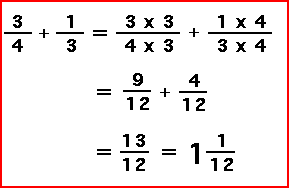To add two or more fractions, first make all the fractions have the same denominator. Once the denominators are the same, just add the numerators. Then simplify the answer (put the fraction in lowest terms).

Multiplying Fractions :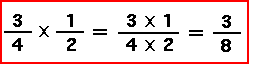To multiply two or more fractions, multiply the numerators and multiply the denominators. Then simplify the answer (put the fraction in lowest terms).

Comparing Fractions :To compare two fractions (that is, to find out which one is bigger or smaller), first write the fractions so that they have the same denominator. Then, compare the numerators; the fraction with the largest positive numerator is the larger fraction.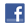Twitter Share
English exercise "Fractions" created by anonyme with The test builder.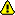Please log in to save your progress.

1. What is a fraction ?

2. Which number is called the numerator ?

3. Why the denominator of a fraction can't be equal to zero ?

4. When is a fraction in its simplest form ?

5. What are equivalent fractions ?

6. What is an improper fraction ?

7. How can an improper fraction be written ?

8. How convert a fraction to a decimal ?

9. How two or more fractions can be added ?

10. How can a fraction be expressed as a percentage ?

End of the free exercise to learn English: Fractions
A free English exercise to learn English.
Other English exercises on the same topic : Numbers | All our lessons and exercises